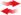Help Home

Weapon Scr. I
Weapon Scr. II
Missions

All Commands
General
Graphics
HUD
Sounds
Player
Terrain
Projectiles
Particles
Collision
Objects
AI
Nodes
Missions

# Commands: terrain

## Legend

• X - X is a number
• 'X' - X is a string (a letter/word/text)
• [X=0] - X is an optional parameter with value 0 when omitted (NEVER add [] to your script!)
•- command is hard synced

### getterraincolor(X, Y, [FORMAT=0])

 Parameters: X - x coordinate Y - y coordinate [FORMAT=0] - result format (0=a,r,g,b integers, 1=argb in one integer)
 Returns: 4 Integers (each 0-255): Alpha,Red,Green,Blue OR 1 Integer containing all values
Gets the color of the terrain at a certain coordinate.

The result can be returned as 4 integers or as a one integer which contains all 4 values (depending on FORMAT).
Use the one integer format if you want to use the color with commands like terrainrect or terraincircle.

ATTENTION: This color value is not necessarily the same on each client (due to random effects like blood and craters)! Do not use it for important conditions. Use it for visual effects only!

###terrainalphaimage(IMAGE, X, Y, [ALPHA=1.0], [RED=-1], [GREEN=-1], [BLUE=-1], [ROTATION=0])

 Parameters: IMAGE - ID of an image X - x coordinate Y - y coordinate [ALPHA=1.0] - alpha value [RED=-1] - use another red value [GREEN=-1] - use another green value [BLUE=-1] - use another blue value [ROTATION=0] - rotation angle?
Mixes IMAGE with the terrain at the (X|Y) coordinate. IMAGE then becomes part of the terrain.
The image will only by drawn on pixels which are terrain and the ALPHA value will be used.
Use the RED, GREEN and BLUE parameters to influence the color. -1 means that the original colors will be used. Values between 0 and 255 mean that these colors will be used instead.
Change ROTATION to draw the image rotated. Attention: Rotating the image is slow and reduces the quality!

###terrainbackimage(IMAGE, X, Y, [RED=0], [GREEN=0], [BLUE=0], [ROTATION=0])

 Parameters: IMAGE - ID of an image X - x coordinate Y - y coordinate [RED=0] - modify red value [GREEN=0] - modify green value [BLUE=0] - modify blue value [ROTATION=0] - rotation angle?
Draws IMAGE on the terrain at the (X|Y) coordinate. IMAGE then becomes part of the terrain.
The image will only by drawn on pixels which are sky (looks like it is drawn in the background).
Use the RED, GREEN and BLUE parameters to influence the color. These values will be added to the image pixel colors. 0 means no modification.
Change ROTATION to draw the image rotated. Attention: Rotating the image is slow and reduces the quality!

###terraincircle(X, Y, RADIUS, COLOR)

 Parameters: X - x coordinate Y - y coordinate RADIUS - radius of the circle COLOR - color (0xAARRGGBB)
Draws a circle. The (X|Y) coordinate is the center of the circle.

COLOR is a hexadecimal number with
0xAARRGGBB = alpha (sky/terrain)
0xAARRGGBB = red
0xAARRGGBB = green
0xAARRGGBB = blue

You can use values from 00 (0) to FF (255). For the alpha value please only use 00 (sky) or FF (terrain).

Commonly COLOR 0x00000000 is used to erase the the terrain.

###terrainexplosion(X, Y, RADIUS, [EFFECT=0])

 Parameters: X - x coordinate Y - y coordinate RADIUS - explosion radius [EFFECT=0] - effect type
Destroys the terrain at the (X|Y) coordinate and creates some effects and sounds.

Available effects are:
0: gun impact
1: explosion
2: digging
3: explosion fx without terrain destruction (FX equals 1)

###terrainimage(IMAGE, X, Y, [IGNORE MASK=0], [ROTATION=0])

 Parameters: IMAGE - ID of an image X - x coordinate Y - y coordinate [IGNORE MASK=0] - ignore mask? [ROTATION=0] - rotation angle
Draws IMAGE into the terrain at the (X|Y) coordinate. IMAGE then becomes part of the terrain.
Use IGNORE MASK 1 if you want to draw the full image including the masked areas / alpha channel.
Change ROTATION to draw the image rotated. Attention: Rotating the image is slow and reduces the quality!

### terrainmodification()

 Returns: MODIFICATION - 1 if a terrain modification occurred recently, else 0
Returns a 1 if the terrain shape has been modified recently, otherwise 0.

###terrainrect(X, Y, WIDTH, HEIGHT, COLOR)

 Parameters: X - x coordinate Y - y coordinate WIDTH - width HEIGHT - height COLOR - color (0xAARRGGBB)
Draws an rectangle. The (X|Y) coordinate is the upper left corner of the rectangle.

COLOR is a hexadecimal number with
0xAARRGGBB = alpha (sky/terrain)
0xAARRGGBB = red
0xAARRGGBB = green
0xAARRGGBB = blue

You can use values from 00 (0) to FF (255). For the alpha value please only use 00 (sky) or FF (terrain).

Commonly COLOR 0x00000000 is used to erase the the terrain.